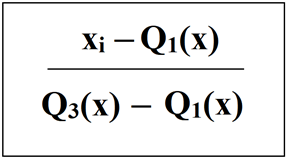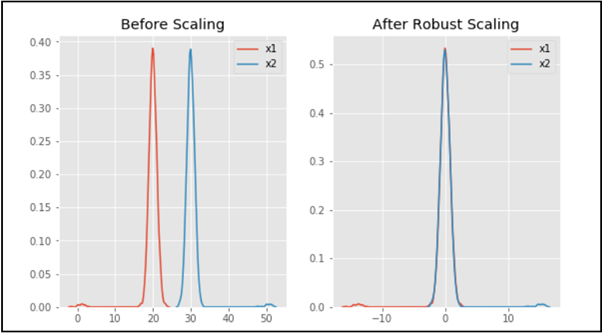# Feature Scaling – Part 3

Prerequisite – Feature Scaling | Set-1 , Set-2

Feature Scaling is one of the most important steps of Data Preprocessing. It is applied to independent variables or features of data. The data sometimes contains features with varying magnitudes and if we do not treat them, the algorithms only take in the magnitude of these features, neglecting the units. It helps to normalize the data in a particular range and sometimes also helps in speeding up the calculations in an algorithm.

#### Robust Scaler:

RobustScaler is a characteristic scaling technique used in gadget learning and statistics analysis this is designed to be robust to outliers. It is a       method used to scale numerical functions in a dataset by way of remodeling them to a commonplace scale, whilst being much less sensitive to the presence of outliers compared to different scaling strategies.

The principal idea at the back of RobustScaler is to use statistical measures which are much less suffering from outliers, including median and interquartile range (IQR), in place of suggest and fashionable deviation utilized in different scaling strategies like StandardScaler. Here are some     key characteristics and benefits of RobustScaler:

1. Robust to outliers: RobustScaler is much less prompted through outliers within the data, because it makes use of median and IQR, which aren’t stricken by severe values. This makes it suitable for datasets with high variability or presence of outliers, where other scaling techniques may additionally deliver biased effects.
2. Resistant to facts distribution: RobustScaler does no longer make any assumptions approximately the underlying facts distribution, making it more versatile and applicable to special kinds of facts.
3. Preserves facts integrity: RobustScaler preserves the rank and order of the records, because it makes use of rank-based total statistics like median, making it appropriate for ordinal data or information with significant ordinal relationships.
4. Handles skewed facts: RobustScaler can take care of skewed facts well, as it’s far based totally on percentiles (median and IQR) which be less stricken by severe values, making it suitable for datasets with skewed distributions.

The formula below is used:Robust Scaler:

Some more properties of Robust Scaler are:

The Robust Scaler is a sort of statistics normalization method utilized in device gaining knowledge of and facts analysis. In addition to the properties stated in Step 2. Three of the original communication, here are a few extra homes of the Robust Scaler:

1. Robust Scaler is proof against outliers: The Robust Scaler is designed to be sturdy to outliers inside the facts. It uses the median and interquartile variety (IQR) to scale the facts, which makes it much less sensitive to excessive values compared to other scaling techniques like         Standard Scaler.
2. Preserves the form of the distribution: The Robust Scaler preserves the form of the distribution with the aid of scaling the statistics using the median and IQR. It does now not assume that the records is commonly disbursed, which makes it suitable for scaling non-Gaussian allotted facts.
3. Scales the statistics among a specified variety: The Robust Scaler scales the information among a unique range, that is determined via the      `quantile_range` parameter. By default, the `quantile_range` is about to `(25.Zero, 75. Zero)`, this means that the records is scaled among the             25th and 75th percentile of the records.
4. Can deal with sparse matrices: The Robust Scaler also can handle sparse matrices, which is useful when working with excessive-dimensional datasets that have many capabilities.
5. Computes the scaling parameters on the training set handiest: Similar to other normalization techniques, the Robust Scaler computes the scaling parameters at the training set most effectively and applies them to the check set. This guarantees that the take-a-look-at set isn’t used to compute the scaling parameters, which can introduce bias inside the assessment of the version’s overall performance.

Code:

## Python3

 `# Python code for Feature Scaling using Robust Scaling`   `""" PART 1: Importing Libraries """` `import` `pandas as pd` `import` `numpy as np` `from` `sklearn ``import` `preprocessing` `import` `matplotlib` `import` `matplotlib.pyplot as plt` `import` `seaborn as sns ``%` `matplotlib inline` `matplotlib.style.use(``'ggplot'``)`   `""" PART 2: Making the data distributions """`   `x ``=` `pd.DataFrame({` `  `  `    ``# Distribution with lower outliers` `    ``'x1'``: np.concatenate([np.random.normal(``20``, ``1``, ``2000``), np.random.normal(``1``, ``1``, ``20``)]),` `    ``# Distribution with higher outliers` `    ``'x2'``: np.concatenate([np.random.normal(``30``, ``1``, ``2000``), np.random.normal(``50``, ``1``, ``20``)]),` `})`     `""" PART 3: Scaling the Data """` `scaler ``=` `preprocessing.RobustScaler()` `robust_scaled_df ``=` `scaler.fit_transform(x)` `robust_scaled_df ``=` `pd.DataFrame(robust_scaled_df, columns``=``[``'x1'``, ``'x2'``])`   `""" PART 4: Visualizing the impact of scaling """` `fig, (ax1, ax2, ax3) ``=` `plt.subplots(ncols``=``3``, figsize``=``(``9``, ``5``))` `ax1.set_title(``'Before Scaling'``)` `sns.kdeplot(x[``'x1'``], ax``=``ax1)` `sns.kdeplot(x[``'x2'``], ax``=``ax1)` `ax2.set_title(``'After Robust Scaling'``)` `sns.kdeplot(robust_scaled_df[``'x1'``], ax``=``ax2)` `sns.kdeplot(robust_scaled_df[``'x2'``], ax``=``ax2)`

Output:As you can see in the output, after Robust scaling, the distributions are brought into the same scale and overlap, but the outliers remain outside of the bulk of the new distributions. Thus, Robust scaling is an effective method of scaling the data.

Whether you're preparing for your first job interview or aiming to upskill in this ever-evolving tech landscape, GeeksforGeeks Courses are your key to success. We provide top-quality content at affordable prices, all geared towards accelerating your growth in a time-bound manner. Join the millions we've already empowered, and we're here to do the same for you. Don't miss out - check it out now!

Previous
Next